# Graph Theory - Matchings

Advertisements

A matching graph is a subgraph of a graph where there are no edges adjacent to each other. Simply, there should not be any common vertex between any two edges.

## Matching

Let ‘G’ = (V, E) be a graph. A subgraph is called a matching M(G), if each vertex of G is incident with at most one edge in M, i.e.,

deg(V) ≤ 1 ∀ V ∈ G

which means in the matching graph M(G), the vertices should have a degree of 1 or 0, where the edges should be incident from the graph G.

Notation − M(G)

Example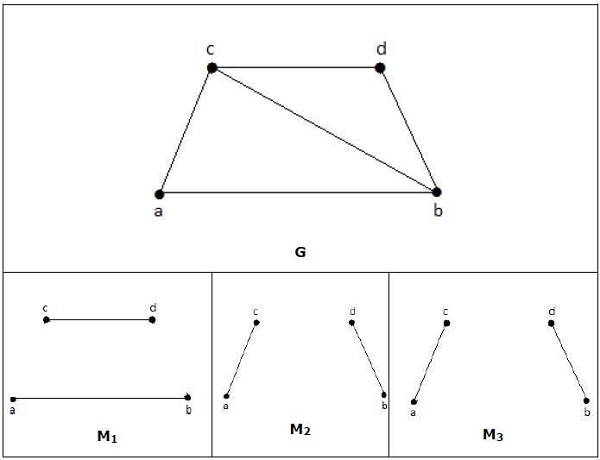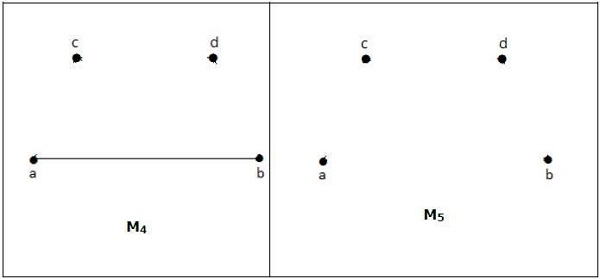In a matching,

if deg(V) = 1, then (V) is said to be matched

if deg(V) = 0, then (V) is not matched.

In a matching, no two edges are adjacent. It is because if any two edges are adjacent, then the degree of the vertex which is joining those two edges will have a degree of 2 which violates the matching rule.

## Maximal Matching

A matching M of graph ‘G’ is said to maximal if no other edges of ‘G’ can be added to M.

Example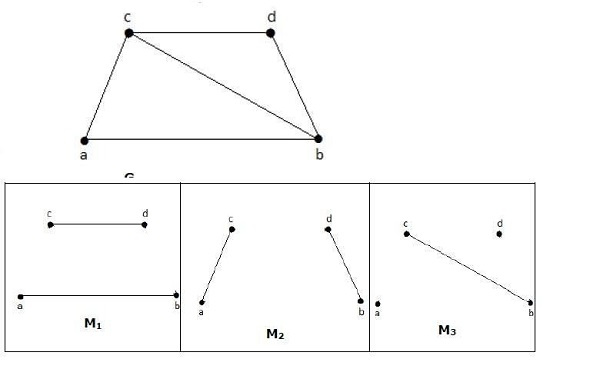M1, M2, M3 from the above graph are the maximal matching of G.

## Maximum Matching

It is also known as largest maximal matching. Maximum matching is defined as the maximal matching with maximum number of edges.

The number of edges in the maximum matching of ‘G’ is called its matching number.

Example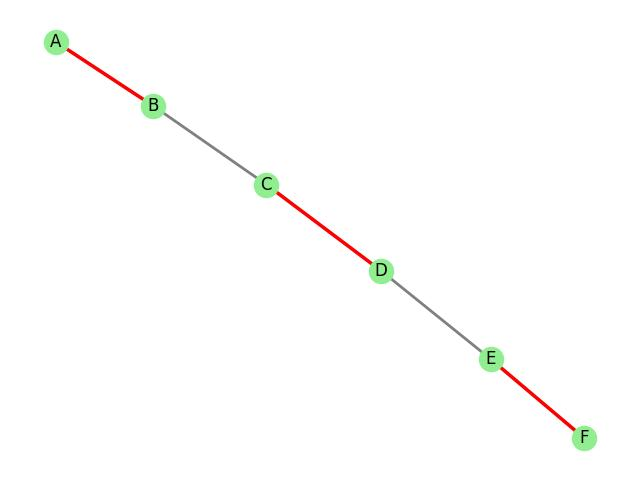For a graph given in the above example, M1 and M2 are the maximum matching of ‘G’ and its matching number is 2. Hence by using the graph G, we can form only the subgraphs with only 2 edges maximum. Hence we have the matching number as two.

## Perfect Matching

A matching (M) of graph (G) is said to be a perfect match, if every vertex of graph g (G) is incident to exactly one edge of the matching (M), i.e.,

deg(V) = 1 ∀ V

The degree of each and every vertex in the subgraph should have a degree of 1.

Example

In the following graphs, M1 and M2 are examples of perfect matching of G.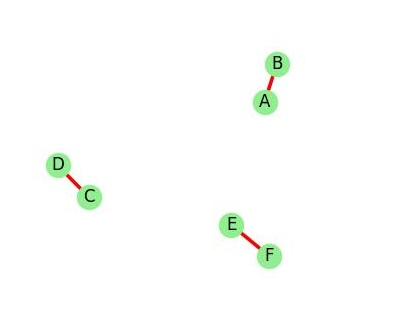Note − Every perfect matching of graph is also a maximum matching of graph, because there is no chance of adding one more edge in a perfect matching graph.

A maximum matching of graph need not be perfect. If a graph ‘G’ has a perfect match, then the number of vertices |V(G)| is even. If it is odd, then the last vertex pairs with the other vertex, and finally there remains a single vertex which cannot be paired with any other vertex for which the degree is zero. It clearly violates the perfect matching principle.

Example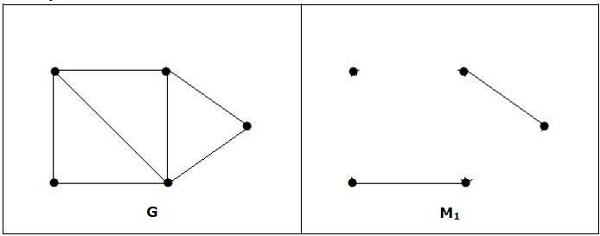Note − The converse of the above statement need not be true. If G has even number of vertices, then M1 need not be perfect.

Example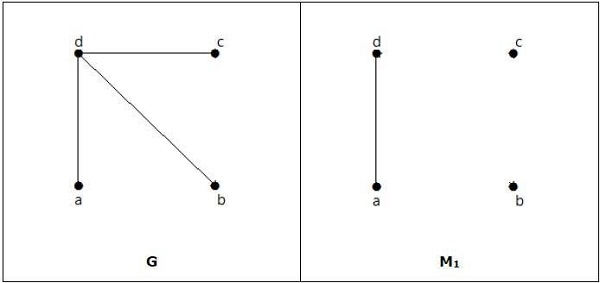It is matching, but it is not a perfect match, even though it has even number of vertices.

Advertisements